# Linear regression¶

This note is to remind myself about some of the basic concepts of linear regression.

Bibliography

 James, Witten, Hastie, Tibshirani - Introduction to Statistical Learning

## Setup¶

In :
import numpy as np
import scipy.stats
import pandas as pd
import matplotlib.pylab as plt

In :
%matplotlib inline

In :
(blue, orange, red, green, purple, brown, pink, yellow, lightred, lightblue,
lightorange, lightgreen, lightpurple) = \
('#377eb8', '#ff7f00', '#e41a1c', '#4daf4a', '#984ea3', '#a65628', '#f781bf',
'#d2d215', '#fb9a99', '#a6cee3', '#fdbf6f', '#b2df8a', '#cab2d6')


## Creating some (noisy) toy data¶

In :
def create_data(b0, b1, noise_sd, n=100):
"""Return a dataframe with n rows and columns 'X' and 'Y'
The dataframe will contain random data points that follow
Y = b1 * X + b0 + eps where the error eps is drawn from
a normal distribution with standard deviation noise_sd
"""
x = np.sort(10 * np.random.rand(n))
y = b1 * x + b0
noise = scipy.stats.norm(scale=noise_sd)
y += noise.rvs(len(x))
return pd.DataFrame({'X': x, 'Y': y})

In :
b0_true = 0.3
b1_true = 1.2
noise_sd = 2.0  # σ in the standard distribution

data = create_data(b0_true, b1_true, noise_sd)

def plot_data(data, *lines, show=True, **kwargs):
fig, ax = plt.subplots(**kwargs)
data.plot.scatter('X', 'Y', ax=ax)
for (b0, b1, label, color) in lines:
ax.plot(
np.linspace(0, 10, 5),
b0 + np.linspace(0, 10, 5) * b1,
color=color, label=label)
ax.legend()
if show:
plt.show(fig)
else:
return fig, ax

plot_data(data, (b0_true, b1_true, 'true line', 'red'))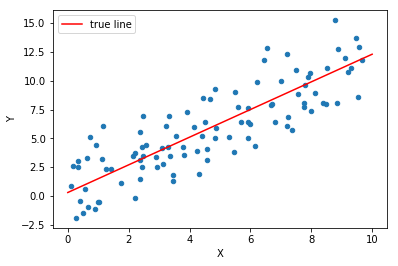## Linear Regression with statsmodels¶

In :
import statsmodels.api as sm

/Users/goerz/anaconda3/lib/python3.5/site-packages/statsmodels/compat/pandas.py:56: FutureWarning: The pandas.core.datetools module is deprecated and will be removed in a future version. Please use the pandas.tseries module instead.
from pandas.core import datetools

In :
mod = sm.OLS(data['Y'], sm.add_constant(data['X']))

In :
res = mod.fit()
res.summary()

Out:
Dep. Variable: R-squared: Y 0.710 OLS 0.707 Least Squares 239.8 Tue, 02 Jan 2018 4.39e-28 14:38:36 -213.37 100 430.7 98 436.0 1 nonrobust
coef std err t P>|t| [0.025 0.975] 0.5642 0.396 1.424 0.158 -0.222 1.350 1.1292 0.073 15.486 0.000 0.984 1.274
 Omnibus: Durbin-Watson: 5.086 2.139 0.079 3.69 0.332 0.158 2.332 10.7
In :
res.params

Out:
const    0.564158
X        1.129185
dtype: float64

## Manual Linear Regression¶

In :
def linear_regression(data):
"""Estimated b0_hat, b1_hat for the given data.

See  Eq. (3.4)
"""
xbar = data['X'].mean()
ybar = data['Y'].mean()
delta_x = data['X'] - xbar
delta_y = data['Y'] - ybar
b1_hat = np.dot(delta_x, delta_y) / np.dot(delta_x, delta_x)
b0_hat = ybar - b1_hat * xbar
return b0_hat, b1_hat

In :
b0_hat, b1_hat = linear_regression(data)

In :
b0_hat, b1_hat

Out:
(0.56415800312160069, 1.1291854795890905)
In :
b0_true, b1_true

Out:
(0.3, 1.2)
In :
plot_data(
data,
(b0_true, b1_true, 'true line ("population regression line")', red),
(b0_hat, b1_hat, 'fit ("least squares line")', blue)
)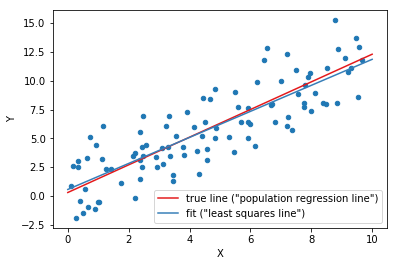## Goodness of Fit¶

### Standard Error¶

The Standard Error describes how close an estimated quantity is expected to be to the actual quantity.

It is the standard deviation of the "sampling distribution" of a statistical quantity: If you take a large number of independent samples, each of size $n$, and calculate the statistical quantity for each sample individually, then the standard errror is the standard deviation of the distribution of those results. It is always $\sigma/\sqrt{n}$, where $\sigma$ is the (possibly unknown) standard deviation of the original distribution. Standard errors go to zero as sample sizes go to infinity; standard deviations do not.

In :
def residuals(data, b0, b1):
"""Array of residuals from the perfect linear relation Y=b1*X+b0"""
Y_hat = b1 * data['X'] + b0
return data['Y'] - Y_hat

In :
def RSS(data, b0, b1):
"""Residual Sum of Squares

How much the Y values vary around the predicted Y values
"""
return (residuals(data, b0, b1)**2).sum()

In :
def TSS(data):
"""Total Sum of Squares

How much the Y values vary around the mean Y value
"""
return ((data['Y'] - data['Y'].mean())**2).sum()

In :
def RSE(data, b0, b1):
"""Residual standard error

This is an estimate for the standard deviation σ of the true
distribution (noise_sd). The y-values in the sample deviate from the
from the true regression line by about RSE units on average.
"""
return np.sqrt(RSS(data, b0, b1) / (len(data)-2))

In :
RSS(data, b0_hat, b1_hat)

Out:
417.67279110404758
In :
RSE(data, b0_hat, b1_hat)

Out:
2.0644532584109894
In :
noise_sd

Out:
2.0
In :
def linear_regression_SE(data, sig):
"""Calculate the standard error for the estimate of b0, b1 in a
linear regression for the given data, assuming a standard deviation of
sig for the distribution of noise on Y. As sig is generally not known,
it should be estimated by the RSE.

Note that the standard error is a property of the sampling only:
The Y-values do not enter!

See  Eq. (3.8)
"""
n = len(data)
xbar = data['X'].mean()
delta_x = data['X'] - xbar
denom = np.dot(delta_x, delta_x)
se_b0_sq = sig**2 * (1.0 / n + xbar**2/denom)
se_b1_sq = sig**2 / denom
return np.sqrt(se_b0_sq), np.sqrt(se_b1_sq)

In :
linear_regression_SE(data, RSE(data, b0_hat, b1_hat))

Out:
(0.39617022407597224, 0.072915570947221814)

The 95% confidence intervals is $\pm$ two standard errors (probability that the true value is in the range of the confidence interval)

### Confidence Interval plot¶

In :
def regline_SE(data, sig, xgrid):
"""The standard error of the full regression line, assuming
a standard deviation of sig for the distribution of noise on Y. As
sig is generally not known, it should be estimated by the RSE.
"""
n = len(data)
return sig * np.sqrt(
(1.0 / n) + (
(xgrid - data['X'].mean())**2 /
((data['X'] - data['X'].mean())**2).sum()))


The 95% confidence band can be calculated as:

In :
def regline_confidence_bands(data, b0_hat, b1_hat, xgrid, confidence=0.95):
"""Return two lines (arrays of y-values), the lower and upper boundary
of the confidence band"""
Y_hat = b0_hat + b1_hat * xgrid  # the fitted line, on the given xgrid
n = len(data)
sig = RSE(data, b0_hat, b1_hat)
se = regline_SE(data, sig, xgrid)
t_minus, t_plus = scipy.stats.t(df=n-2).interval(confidence)
return Y_hat + t_minus * se, Y_hat + t_plus * se

In :
fig, ax = plot_data(
data,
(b0_true, b1_true, 'true line ("population regression line")', red),
(b0_hat, b1_hat, 'fit ("least squares line")', blue),
show=False, figsize=(10, 6)
)
xgrid = np.linspace(0, 10, 100)
lm, lp = regline_confidence_bands(data, b0_hat, b1_hat, xgrid)
ax.fill_between(
xgrid, lm, lp,
color='black', alpha=0.2, label='95% confidence band')
ax.legend()
plt.show(fig)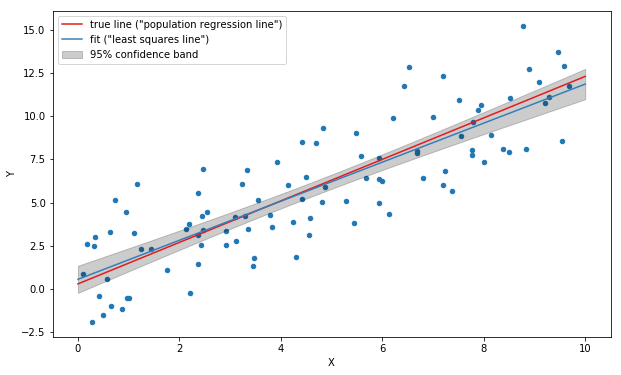The confidence band should be interpreted as follows: There is a 95% probability that a fit based on any given sampling of the same distribution will land in the interval.

### Testing the null hypothesis¶

The null-hypthesis is that Y and X have no reltionship, i.e. b1 = 0. The $t$-value is the b1 in units of the standard error:

In :
t = b1_hat / linear_regression_SE(data, RSE(data, b0_hat, b1_hat))
t

Out:
15.48620500285768

The $t$-value follows a student distribution, and we can calculate a p-value (probability that $t$ has the given value, instead of 0)

In :
2 * scipy.stats.t(df=len(data)-2).sf(t)  # = 1 - (cdf(t) - cdf(-t))

Out:
4.387286482055839e-28

### The $R^2$ statistic¶

In :
def R_sq(data, b0, b1):
"""R^2 in [0, 1] measures how well the variation in Y around the mean
matches the variation in Y around the predicted value.

That is, how much of the variability can be explained by the sampling"""
return 1 - RSS(data, b0, b1) / TSS(data)

In :
R_sq(data, b0_hat, b1_hat)

Out:
0.70990686874758846

A value close to 1 means that the linear fit is very good

### Normality of the residuals¶

A graphical way to check that the residuals are normal-distributed is to plot the quantiles of the residules against the quantiles of a normal distribution, see https://stats.stackexchange.com/questions/321061/probability-that-residuals-are-normal/321071#321071

In :
fig, ax = plt.subplots()
ax.plot(
scipy.stats.norm().ppf(np.linspace(0, 1, len(data))),
residuals(data, b0_hat, b1_hat).sort_values() / RSE(data, b0_hat, b1_hat))
ax.set_title('QQ plot')
ax.plot(
np.linspace(-3, 3, 10), np.linspace(-3, 3, 10), color='black',
ls='dashed')
ax.set_xlabel('theoretical quantiles')
ax.set_ylabel('sample quantiles')
plt.show(fig)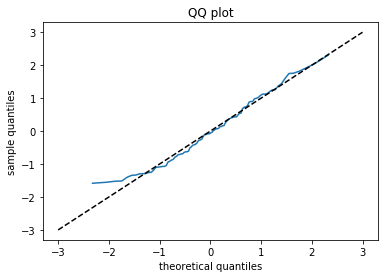## Fit with known slope¶

In :
b0_known_slope = np.mean(data['Y'] - b1_true*data['X'])

In :
b0_known_slope

Out:
0.23577220342661306
In :
b0_true

Out:
0.3
In :
plot_data(
data,
(b0_true, b1_true, 'true line', red),
(b0_hat, b1_hat, 'fit', blue),
(b0_known_slope, b1_true, 'fit with known slope', orange),
show=True,
)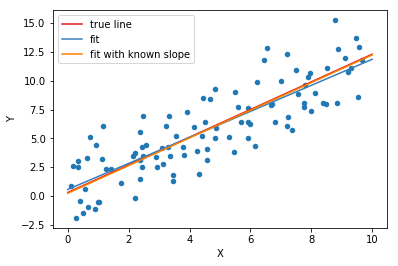In [ ]: